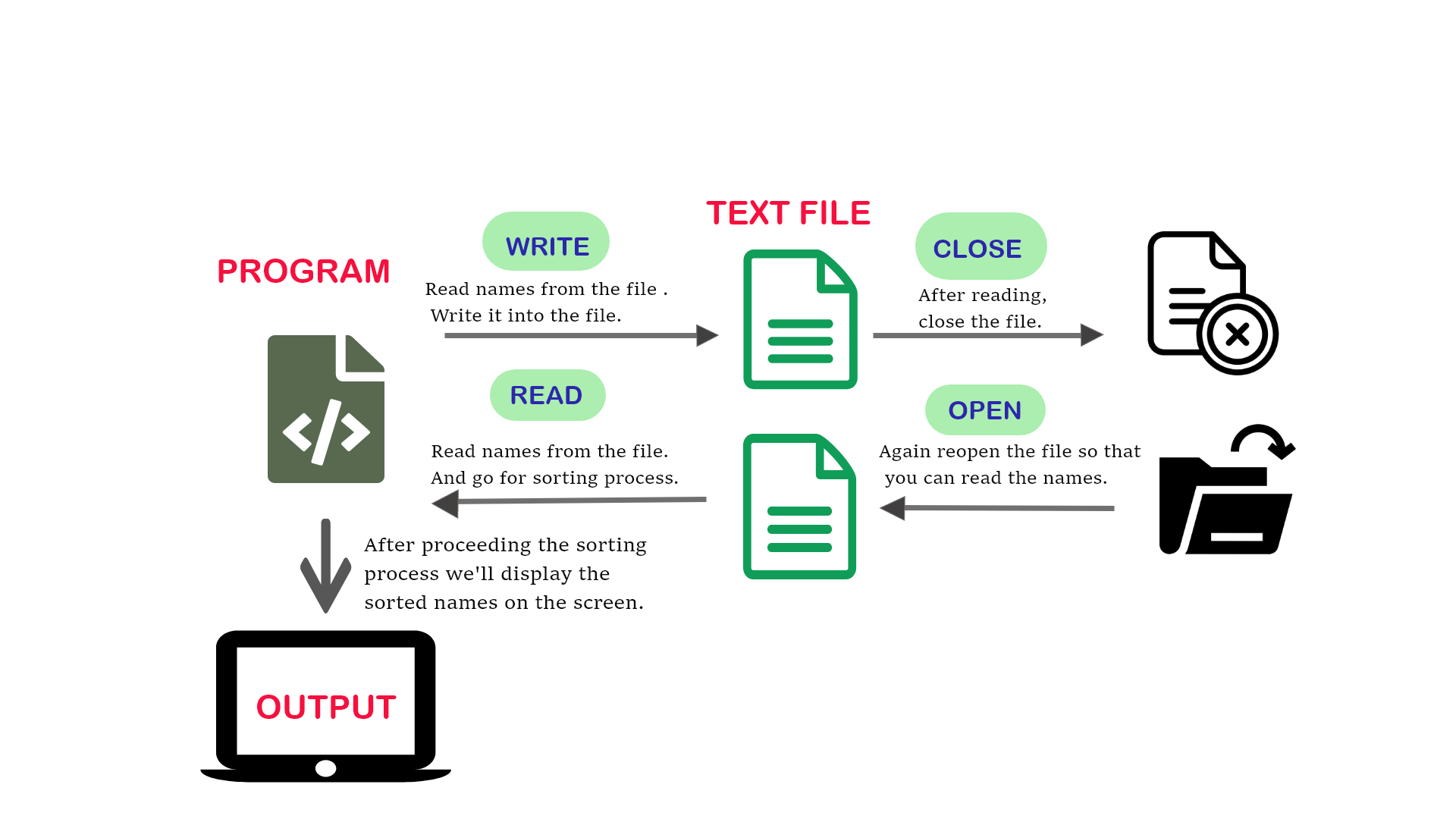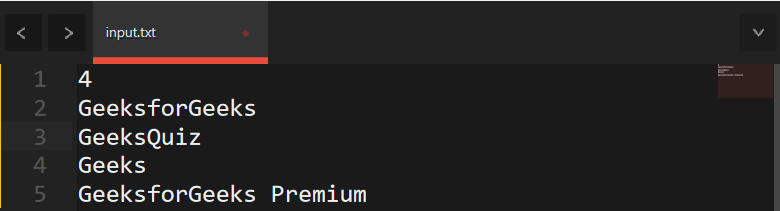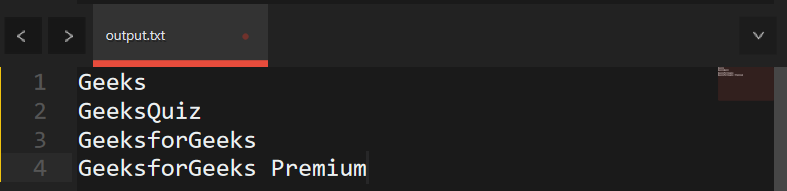GeeksforGeeks App
Open AppBrowser
Continue

# Sorting strings from the text file

Given a text file “file.txt” that consists of strings, the task is to sort all the strings in alphabetical order in that text file.## Recommended: Please try your approach on {IDE} first, before moving on to the solution.

Approach: The idea is to use the concept of File Handling and a text file(say file.txt) that contains all the strings. Below are the steps:

Below is the implementation of the above approach:

## C++

 `// C++ program to sort given array``// of string stored in a file``#include ``#include ``#include ``#include ``using` `namespace` `std;` `// Driver Code``int` `main()``{``    ``int` `N, i, j;` `    ``// File pointer to open file``    ``FILE``* f;` `    ``// fopen() for creating of a file``    ``f = ``fopen``(``"file.txt"``, ``"w"``);` `    ``// Input number of strings``    ``// to be inserted in file``    ``cin >> n;` `    ``vector<``int``> name(N);` `    ``// Insert the strings into file``    ``for` `(i = 0; i < n; i++) {` `        ``// Insert names in file``        ``cin >> name[i];` `        ``// Writing into the file``        ``fprintf``(f, ``"%s"``, name[i]);``    ``}` `    ``// Close the file``    ``fclose``(f);` `    ``// Reopening in read mode``    ``f = ``fopen``(``"file.txt"``, ``"r"``);` `    ``// Check does file exist or not``    ``if` `(f == NULL) {``        ``cout << ``"File doesn't exist!"``;``        ``return` `0;``    ``}` `    ``// Read the file until it``    ``// encounters end of line``    ``while` `(!``feof``(f)) {``        ``fscanf``(f, ``"%s"``, name[i]);``        ``i++;``    ``}``    ``n = i - 1;` `    ``// Sort the strings``    ``sort(name.begin(), name.end());` `    ``// Insert the strings into file``    ``// after sorting``    ``for` `(i = 0; i < n; i++) {` `        ``// Write into the file``        ``fprintf``(f, ``"%s"``, name[i]);``    ``}` `    ``// Print the sorted names``    ``for` `(i = 0; i < n; i++) {``        ``cout << name[i] << ``'\n'``;``    ``}` `    ``return` `0;``}`

## Java

 `import` `java.io.BufferedReader;``import` `java.io.BufferedWriter;``import` `java.io.FileReader;``import` `java.io.FileWriter;``import` `java.io.IOException;``import` `java.util.ArrayList;``import` `java.util.Collections;``import` `java.util.List;``import` `java.util.Scanner;` `public` `class` `SortStringsFromFile {``    ``public` `static` `void` `main(String[] args) {``        ``Scanner scanner = ``new` `Scanner(System.in);` `        ``// Input number of strings to be inserted in file``        ``System.out.print(``"Enter the number of strings: "``);``        ``int` `n = scanner.nextInt();``        ``scanner.nextLine();` `        ``try` `{``            ``// Open file for writing``            ``BufferedWriter writer = ``new` `BufferedWriter(``new` `FileWriter(``"file.txt"``));` `            ``// Insert the strings into file``            ``for` `(``int` `i = ``0``; i < n; i++) {``                ``System.out.print(``"Enter the string: "``);``                ``String name = scanner.nextLine();` `                ``// Writing into the file``                ``writer.write(name + ``"\n"``);``            ``}` `            ``// Close the writer``            ``writer.close();` `            ``// Open file for reading``            ``BufferedReader reader = ``new` `BufferedReader(``new` `FileReader(``"file.txt"``));` `            ``// Read the lines until end of file is reached``            ``List names = ``new` `ArrayList();``            ``String line;``            ``while` `((line = reader.readLine()) != ``null``) {``                ``names.add(line);``            ``}` `            ``// Close the reader``            ``reader.close();` `            ``// Sort the strings``            ``Collections.sort(names);` `            ``// Open the file for writing``            ``writer = ``new` `BufferedWriter(``new` `FileWriter(``"file.txt"``));` `            ``// Insert the sorted strings into the file``            ``for` `(String name : names) {``                ``writer.write(name + ``"\n"``);``            ``}` `            ``// Close the writer``            ``writer.close();` `            ``// Print the sorted names``            ``for` `(String name : names) {``                ``System.out.println(name);``            ``}` `        ``} ``catch` `(IOException e) {``            ``e.printStackTrace();``        ``}` `        ``scanner.close();``    ``}``}`

## Python3

 `# Python program to sort given array of string stored in a file` `# Input number of strings to be inserted in file``N ``=` `int``(``input``(``"Enter the number of strings: "``))` `# Open file for writing``with ``open``(``"file.txt"``, ``"w"``) as f:``    ``# Insert the strings into file``    ``for` `i ``in` `range``(N):``        ``name ``=` `input``(``"Enter the string: "``)``        ``# Writing into the file``        ``f.write(name ``+` `"\n"``)` `# Open file for reading``with ``open``(``"file.txt"``, ``"r"``) as f:``    ``# Read the lines until end of file is reached``    ``names ``=` `[line.strip() ``for` `line ``in` `f.readlines()]` `# Sort the strings``names.sort()` `# Open the file for writing``with ``open``(``"file.txt"``, ``"w"``) as f:``    ``# Insert the sorted strings into the file``    ``for` `name ``in` `names:``        ``f.write(name ``+` `"\n"``)` `# Print the sorted names``for` `name ``in` `names:``    ``print``(name)`

## C#

 `using` `System;``using` `System.Collections.Generic;``using` `System.IO;` `public` `class` `SortStringsFromFile``{``    ``public` `static` `void` `Main()``    ``{``        ``// Input number of strings to be inserted in file``        ``Console.Write(``"Enter the number of strings: "``);``        ``int` `n = Convert.ToInt32(Console.ReadLine());` `        ``try``        ``{``            ``// Open file for writing``            ``StreamWriter writer = ``new` `StreamWriter(``"file.txt"``);` `            ``// Insert the strings into file``            ``for` `(``int` `i = 0; i < n; i++)``            ``{``                ``Console.Write(``"Enter the string: "``);``                ``string` `name = Console.ReadLine();` `                ``// Writing into the file``                ``writer.WriteLine(name);``            ``}` `            ``// Close the writer``            ``writer.Close();` `            ``// Open file for reading``            ``StreamReader reader = ``new` `StreamReader(``"file.txt"``);` `            ``// Read the lines until end of file is reached``            ``List<``string``> names = ``new` `List<``string``>();``            ``string` `line;``            ``while` `((line = reader.ReadLine()) != ``null``)``            ``{``                ``names.Add(line);``            ``}` `            ``// Close the reader``            ``reader.Close();` `            ``// Sort the strings``            ``names.Sort();` `            ``// Open the file for writing``            ``writer = ``new` `StreamWriter(``"file.txt"``);` `            ``// Insert the sorted strings into the file``            ``foreach` `(``string` `name ``in` `names)``            ``{``                ``writer.WriteLine(name);``            ``}` `            ``// Close the writer``            ``writer.Close();` `            ``// Print the sorted names``            ``foreach` `(``string` `name ``in` `names)``            ``{``                ``Console.WriteLine(name);``            ``}` `        ``}``        ``catch` `(IOException e)``        ``{``            ``Console.WriteLine(``"Error: "` `+ e.Message);``        ``}` `        ``Console.ReadLine();``    ``}``}`

## Javascript

 `const readline = require(``'readline'``);``const fs = require(``'fs'``);` `const rl = readline.createInterface({``  ``input: process.stdin,``  ``output: process.stdout``});` `// Open file for writing``const f = fs.openSync(``'file.txt'``, ``'w'``);` `rl.question(``'Input number of strings to be inserted in file: '``, (n) => {``  ``let names = [];``  ` `  ``// Insert the strings into file``  ``for` `(let i = 0; i < n; i++) {``    ``rl.question(``'Enter name: '``, (name) => {``      ``names.push(name);``      ``// Writing into the file``      ``fs.writeSync(f, name);``      ``if` `(i !== n - 1) {``        ``fs.writeSync(f, ``'\n'``);``      ``} ``else` `{``        ``// Close the file``        ``fs.closeSync(f);` `        ``// Reopening in read mode``        ``const data = fs.readFileSync(``'file.txt'``, ``'utf8'``).split(``'\n'``);``        ``const len = data.length;``        ``if` `(data[len - 1] === ``''``) {``          ``data.pop();``        ``}` `        ``// Sort the strings``        ``data.sort();` `        ``// Insert the strings into file after sorting``        ``fs.writeFileSync(``'file.txt'``, data.join(``'\n'``));` `        ``// Print the sorted names``        ``console.log(data.join(``'\n'``));``      ``}``    ``});``  ``}``});`

Input File:Output File:My Personal Notes arrow_drop_up